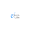## What is the full form of PRNG?

• Pseudo-Random Number Generator

### What does PRNG mean?

A PRNG is an algorithm that generates random number sequences using mathematical formulas. PRNG generates a series of numbers that closely resemble random numbers. A seed state is used to start a PRNG from an arbitrary state. Many numbers are generated in a short amount of time and can be reproduced later if the sequence starting point is known. as a result, the numbers are predictable and efficient.

## What is the full form of PRNG?

• Pseudo-Random Number Generator

### What does PRNG mean?

A PRNG is an algorithm that generates random number sequences using mathematical formulas. PRNG generates a series of numbers that closely resemble random numbers. A seed state is used to start a PRNG from an arbitrary state. Many numbers are generated in a short amount of time and can be reproduced later if the sequence starting point is known. as a result, the numbers are predictable and efficient.

#### 1 comment:

1.